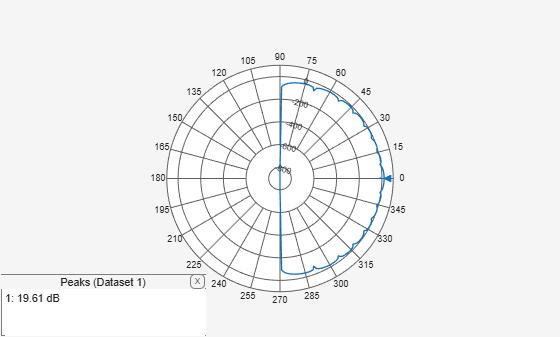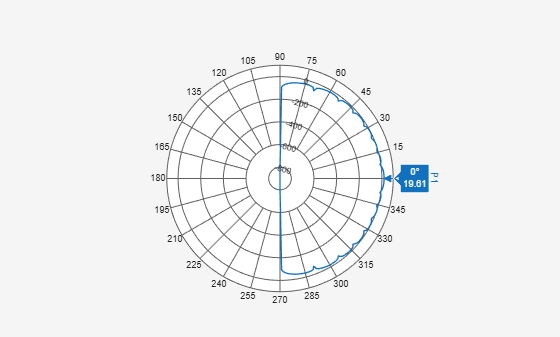# showPeaksTable

Class: polarpattern

Show or hide peak marker table

## Syntax

``showPeaksTable(p,vis)``

## Description

example

````showPeaksTable(p,vis)` shows or hides a table of the peak values. By default, the peak values table is visible.```

## Input Arguments

expand all

Polar plot, specified as a scalar handle.

Show or hide peaks table, specified as `0` or `1`.

## Examples

expand all

Create a 15-element ULA of cosine antennas with elements spaced one-half wavelength apart. Then, plot the directivity of the array at 20 GHz.

Note: This example runs only in R2016b or later. If you are using an earlier release, replace each call to the function with the equivalent `step` syntax. For example, replace `myObject(x)` with `step(myObject,x)`.

```fc = 20.0e9; c = physconst('Lightspeed'); lam = c/fc; angs = [-180:1:180]; antenna = phased.CosineAntennaElement('FrequencyRange',[1.0e9,100.0e9],... 'CosinePower',[2.5 2.5]); array = phased.ULA('Element',antenna,'NumElements',15,'ElementSpacing',lam/2);```

Plot the polar pattern and show three peaks of the antenna. When creating a `polarpattern` plot, if you specify the Peaks property, the peaks table is displayed by default.

```a = pattern(array,fc,angs,0); P = polarpattern(angs,a,'Peaks',3);```Hide the table. When the peaks table is hidden, the peak markers display the peak values.

`showPeaksTable(P,0);`